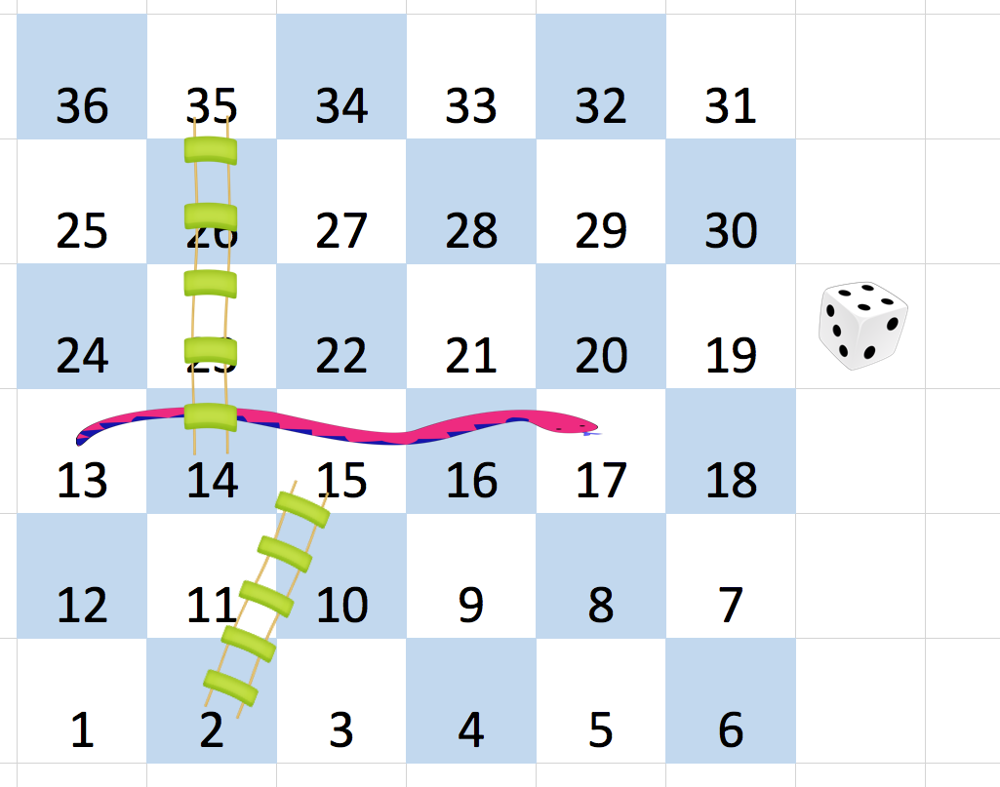# 909. Snakes and Ladders Solution C++

You are given an `n x n` integer matrix `board` where the cells are labeled from `1` to `n2` in a Boustrophedon style starting from the bottom left of the board (i.e. `board[n - 1]`) and alternating direction each row.

You start on square `1` of the board. In each move, starting from square `curr`, do the following:

• Choose a destination square `next` with a label in the range `[curr + 1, min(curr + 6, n2)]`.
• This choice simulates the result of a standard 6-sided die roll: i.e., there are always at most 6 destinations, regardless of the size of the board.
• If `next` has a snake or ladder, you must move to the destination of that snake or ladder. Otherwise, you move to `next`.
• The game ends when you reach the square `n2`.

A board square on row `r` and column `c` has a snake or ladder if `board[r][c] != -1`. The destination of that snake or ladder is `board[r][c]`. Squares `1` and `n2` do not have a snake or ladder.

Note that you only take a snake or ladder at most once per move. If the destination to a snake or ladder is the start of another snake or ladder, you do not follow the subsequent snake or ladder.

• For example, suppose the board is `[[-1,4],[-1,3]]`, and on the first move, your destination square is `2`. You follow the ladder to square `3`, but do not follow the subsequent ladder to `4`.

Return the least number of moves required to reach the square `n2`. If it is not possible to reach the square, return `-1`.

Example 1:```Input: board = [[-1,-1,-1,-1,-1,-1],[-1,-1,-1,-1,-1,-1],[-1,-1,-1,-1,-1,-1],[-1,35,-1,-1,13,-1],[-1,-1,-1,-1,-1,-1],[-1,15,-1,-1,-1,-1]]
Output: 4
Explanation:
In the beginning, you start at square 1 (at row 5, column 0).
You decide to move to square 2 and must take the ladder to square 15.
You then decide to move to square 17 and must take the snake to square 13.
You then decide to move to square 14 and must take the ladder to square 35.
You then decide to move to square 36, ending the game.
This is the lowest possible number of moves to reach the last square, so return 4.
```

Example 2:

```Input: board = [[-1,-1],[-1,3]]
Output: 1
```

Constraints:

• `n == board.length == board[i].length`
• `2 <= n <= 20`
• `grid[i][j]` is either `-1` or in the range `[1, n2]`.
• The squares labeled `1` and `n2` do not have any ladders or snakes.

## Solution -

By reading the question you may have guessed that this can be solved using BFS if you have solved some BFS problems earlier. We will make a queue and then for every possibility, we will check if we can reach the last box or destination. We will also make a visited array so that we do not revisit the same box.
To know which box lead to where we can store it in a map so that we don't iterate in the given matrix again and again.
Here is the implementation

class Solution {
public:
map<int,int>m;
int n=v.size();
int t=0,x=1;
for(int i=n-1; i>=0; i--)
if(t++%2==0)
for(int j=0; j<n; j++)m[x++]=v[i][j];
else
for(int j=n-1; j>=0; j--)m[x++]=v[i][j];
queue<pair<int,int>>q;
q.push({1,0});
vector<int>vis(n*n+1,false);
vis=true;
while(!q.empty()){
pair<int,int>p=q.front();
if(p.first==n*n)return p.second;
q.pop();
for(int i=p.first+1; i<=min(p.first+6,n*n); i++)
if(m[i]==-1 && !vis[i]){q.push({i,p.second+1});vis[i]=true;}
else if(m[i]!=-1 && !vis[m[i]]) {q.push({m[i],p.second+1});vis[m[i]]=true;}
}
return -1;
}
};

Other way without using map, using helper function to get the value from matrix

class Solution {
public:
int m(vector<vector<int>>&v,int d){
int n=v.size();
int row=((d+n-1)/n)-1;
int col=d%n;
if(col!=0)--col;
else col=n-1;
if(row%2==1)col=n-col-1;
return v[row][col];
}

reverse(v.begin(),v.end());
int n=v.size();
int t=0,x=1;
queue<pair<int,int>>q;
q.push({1,0});
vector<int>vis(n*n+1,false);
vis=true;
while(!q.empty()){
pair<int,int>p=q.front();
if(p.first==n*n)return p.second;
q.pop();
for(int i=p.first+1; i<=min(p.first+6,n*n); i++)
if(m(v,i)==-1 && !vis[i]){q.push({i,p.second+1});vis[i]=true;}
else if(m(v,i)!=-1 && !vis[m(v,i)]) {q.push({m(v,i),p.second+1});vis[m(v,i)]=true;}
}
return -1;
}
};